/ clustergram Public

Clustergram - Visualization and diagnostics for cluster analysis in Python

# martinfleis/clustergram

Switch branches/tags
Nothing to show

A tag already exists with the provided branch name. Many Git commands accept both tag and branch names, so creating this branch may cause unexpected behavior. Are you sure you want to create this branch?

## Files

Failed to load latest commit information.
Type
Name
Commit time
January 15, 2023 20:41
January 15, 2023 15:27
February 6, 2023 19:18
January 15, 2023 20:01
January 17, 2023 12:16
January 15, 2023 13:41
May 22, 2023 11:06
January 15, 2023 20:34
October 7, 2020 17:12
January 15, 2023 20:01
January 15, 2023 19:01
January 15, 2023 19:01
January 15, 2023 13:38
January 15, 2023 19:01

# Clustergram## Visualization and diagnostics for cluster analysisClustergram is a diagram proposed by Matthias Schonlau in his paper The clustergram: A graph for visualizing hierarchical and nonhierarchical cluster analyses:

In hierarchical cluster analysis, dendrograms are used to visualize how clusters are formed. I propose an alternative graph called a “clustergram” to examine how cluster members are assigned to clusters as the number of clusters increases. This graph is useful in exploratory analysis for nonhierarchical clustering algorithms such as k-means and for hierarchical cluster algorithms when the number of observations is large enough to make dendrograms impractical.

The clustergram was later implemented in R by Tal Galili, who also gives a thorough explanation of the concept.

This is a Python implementation, originally based on Tal's script, written for `scikit-learn` and RAPIDS `cuML` implementations of K-Means, Mini Batch K-Means and Gaussian Mixture Model (scikit-learn only) clustering, plus hierarchical/agglomerative clustering using `SciPy`. Alternatively, you can create clustergram using `from_*` constructors based on alternative clustering algorithms.## Getting started

You can install clustergram from `conda` or `pip`:

`conda install clustergram -c conda-forge`
`pip install clustergram`

In any case, you still need to install your selected backend (`scikit-learn` and `scipy` or `cuML`).

The example of clustergram on Palmer penguins dataset:

```import seaborn

First we have to select numerical data and scale them.

```from sklearn.preprocessing import scale
data = scale(df.drop(columns=['species', 'island', 'sex']).dropna())```

And then we can simply pass the data to `clustergram`.

```from clustergram import Clustergram

cgram = Clustergram(range(1, 8))
cgram.fit(data)
cgram.plot()```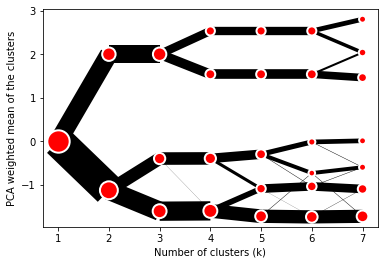## Styling

`Clustergram.plot()` returns matplotlib axis and can be fully customised as any other matplotlib plot.

```seaborn.set(style='whitegrid')

cgram.plot(
ax=ax,
size=0.5,
linewidth=0.5,
cluster_style={"color": "lightblue", "edgecolor": "black"},
line_style={"color": "red", "linestyle": "-."},
figsize=(12, 8)
)```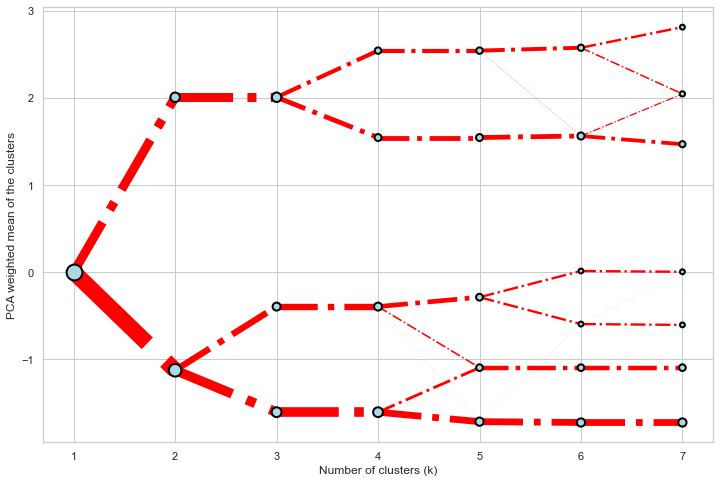## Mean options

On the `y` axis, a clustergram can use mean values as in the original paper by Matthias Schonlau or PCA weighted mean values as in the implementation by Tal Galili.

```cgram = Clustergram(range(1, 8))
cgram.fit(data)
cgram.plot(figsize=(12, 8), pca_weighted=True)```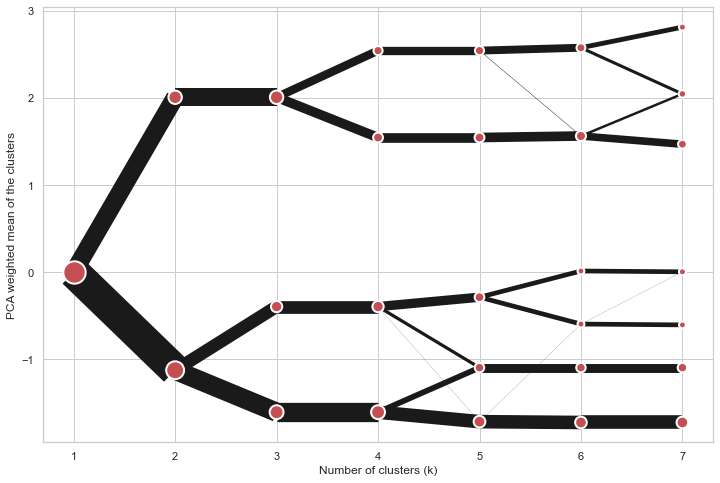```cgram = Clustergram(range(1, 8))
cgram.fit(data)
cgram.plot(figsize=(12, 8), pca_weighted=False)```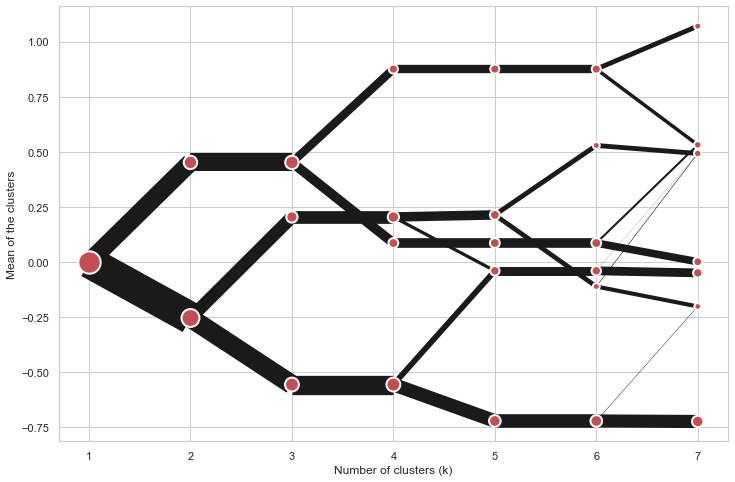## Scikit-learn, SciPy and RAPIDS cuML backends

Clustergram offers three backends for the computation - `scikit-learn` and `scipy` which use CPU and RAPIDS.AI `cuML`, which uses GPU. Note that all are optional dependencies but you will need at least one of them to generate clustergram.

Using `scikit-learn` (default):

```cgram = Clustergram(range(1, 8), backend='sklearn')
cgram.fit(data)
cgram.plot()```

Using `cuML`:

```cgram = Clustergram(range(1, 8), backend='cuML')
cgram.fit(data)
cgram.plot()```

`data` can be all data types supported by the selected backend (including `cudf.DataFrame` with `cuML` backend).

## Supported methods

Clustergram currently supports K-Means, Mini Batch K-Means, Gaussian Mixture Model and SciPy's hierarchical clustering methods. Note tha GMM and Mini Batch K-Means are supported only for `scikit-learn` backend and hierarchical methods are supported only for `scipy` backend.

Using K-Means (default):

```cgram = Clustergram(range(1, 8), method='kmeans')
cgram.fit(data)
cgram.plot()```

Using Mini Batch K-Means, which can provide significant speedup over K-Means:

```cgram = Clustergram(range(1, 8), method='minibatchkmeans', batch_size=100)
cgram.fit(data)
cgram.plot()```

Using Gaussian Mixture Model:

```cgram = Clustergram(range(1, 8), method='gmm')
cgram.fit(data)
cgram.plot()```

Using Ward's hierarchical clustering:

```cgram = Clustergram(range(1, 8), method='hierarchical', linkage='ward')
cgram.fit(data)
cgram.plot()```

## Manual input

Alternatively, you can create clustergram using `from_data` or `from_centers` methods based on alternative clustering algorithms.

Using `Clustergram.from_data` which creates cluster centers as mean or median values:

```data = numpy.array([[-1, -1, 0, 10], [1, 1, 10, 2], [0, 0, 20, 4]])
labels = pandas.DataFrame({1: [0, 0, 0], 2: [0, 0, 1], 3: [0, 2, 1]})

cgram = Clustergram.from_data(data, labels)
cgram.plot()```

Using `Clustergram.from_centers` based on explicit cluster centers.:

```labels = pandas.DataFrame({1: [0, 0, 0], 2: [0, 0, 1], 3: [0, 2, 1]})
centers = {
1: np.array([[0, 0]]),
2: np.array([[-1, -1], [1, 1]]),
3: np.array([[-1, -1], [1, 1], [0, 0]]),
}
cgram = Clustergram.from_centers(centers, labels)
cgram.plot(pca_weighted=False)```

To support PCA weighted plots you also need to pass data:

```cgram = Clustergram.from_centers(centers, labels, data=data)
cgram.plot()```

## Partial plot

`Clustergram.plot()` can also plot only a part of the diagram, if you want to focus on a limited range of `k`.

```cgram = Clustergram(range(1, 20))
cgram.fit(data)
cgram.plot(figsize=(12, 8))```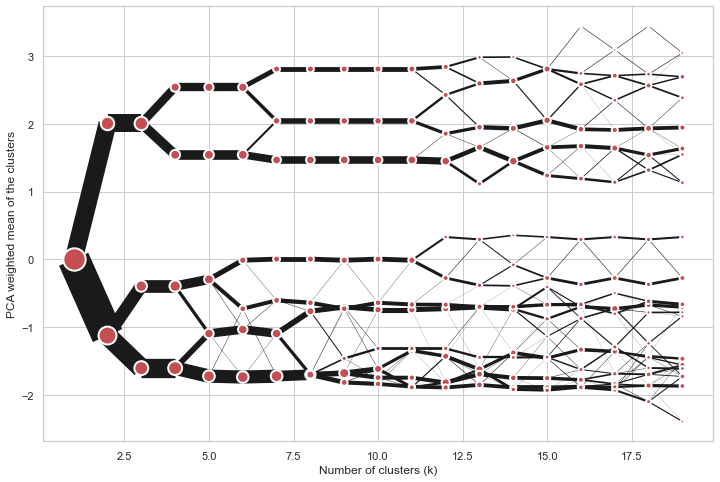`cgram.plot(k_range=range(3, 10), figsize=(12, 8))`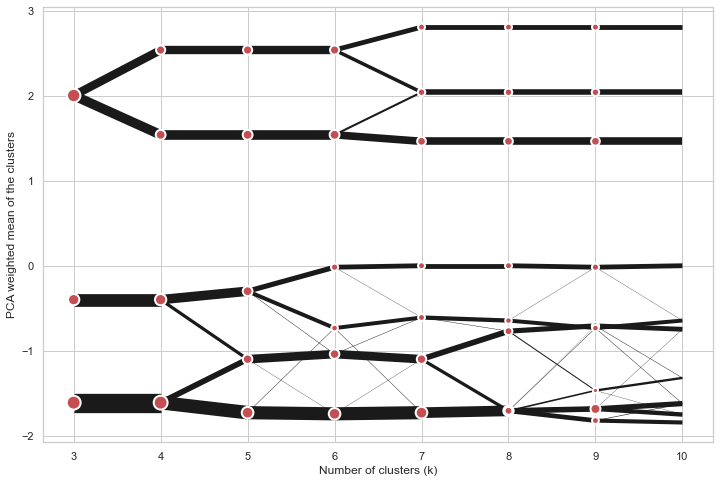Clustergam includes handy wrappers around a selection of clustering performance metrics offered by `scikit-learn`. Data which were originally computed on GPU are converted to numpy on the fly.

### Silhouette score

Compute the mean Silhouette Coefficient of all samples. See `scikit-learn` documentation for details.

```>>> cgram.silhouette_score()
2    0.531540
3    0.447219
4    0.400154
5    0.377720
6    0.372128
7    0.331575
Name: silhouette_score, dtype: float64```

Once computed, resulting Series is available as `cgram.silhouette`. Calling the original method will recompute the score.

### Calinski and Harabasz score

Compute the Calinski and Harabasz score, also known as the Variance Ratio Criterion. See `scikit-learn` documentation for details.

```>>> cgram.calinski_harabasz_score()
2    482.191469
3    441.677075
4    400.392131
5    411.175066
6    382.731416
7    352.447569
Name: calinski_harabasz_score, dtype: float64```

Once computed, resulting Series is available as `cgram.calinski_harabasz`. Calling the original method will recompute the score.

### Davies-Bouldin score

Compute the Davies-Bouldin score. See `scikit-learn` documentation for details.

```>>> cgram.davies_bouldin_score()
2    0.714064
3    0.943553
4    0.943320
5    0.973248
6    0.950910
7    1.074937
Name: davies_bouldin_score, dtype: float64```

Once computed, resulting Series is available as `cgram.davies_bouldin`. Calling the original method will recompute the score.

## Acessing labels

`Clustergram` stores resulting labels for each of the tested options, which can be accessed as:

```>>> cgram.labels
1  2  3  4  5  6  7
0    0  0  2  2  3  2  1
1    0  0  2  2  3  2  1
2    0  0  2  2  3  2  1
3    0  0  2  2  3  2  1
4    0  0  2  2  0  0  3
..  .. .. .. .. .. .. ..
337  0  1  1  3  2  5  0
338  0  1  1  3  2  5  0
339  0  1  1  1  1  1  4
340  0  1  1  3  2  5  5
341  0  1  1  1  1  1  5```

## Saving clustergram

You can save both plot and `clustergram.Clustergram` to a disk.

### Saving plot

`Clustergram.plot()` returns matplotlib axis object and as such can be saved as any other plot:

```import matplotlib.pyplot as plt

cgram.plot()
plt.savefig('clustergram.svg')```

### Saving object

If you want to save your computed `clustergram.Clustergram` object to a disk, you can use `pickle` library:

```import pickle

with open('clustergram.pickle','wb') as f:
pickle.dump(cgram, f)```

```with open('clustergram.pickle','rb') as f:

## References

Schonlau M. The clustergram: a graph for visualizing hierarchical and non-hierarchical cluster analyses. The Stata Journal, 2002; 2 (4):391-402.

Schonlau M. Visualizing Hierarchical and Non-Hierarchical Cluster Analyses with Clustergrams. Computational Statistics: 2004; 19(1):95-111.

https://www.r-statistics.com/2010/06/clustergram-visualization-and-diagnostics-for-cluster-analysis-r-code/

Clustergram - Visualization and diagnostics for cluster analysis in Python

## Releases 9

Version 0.7.0 Latest
Jan 15, 2023

•
•
•